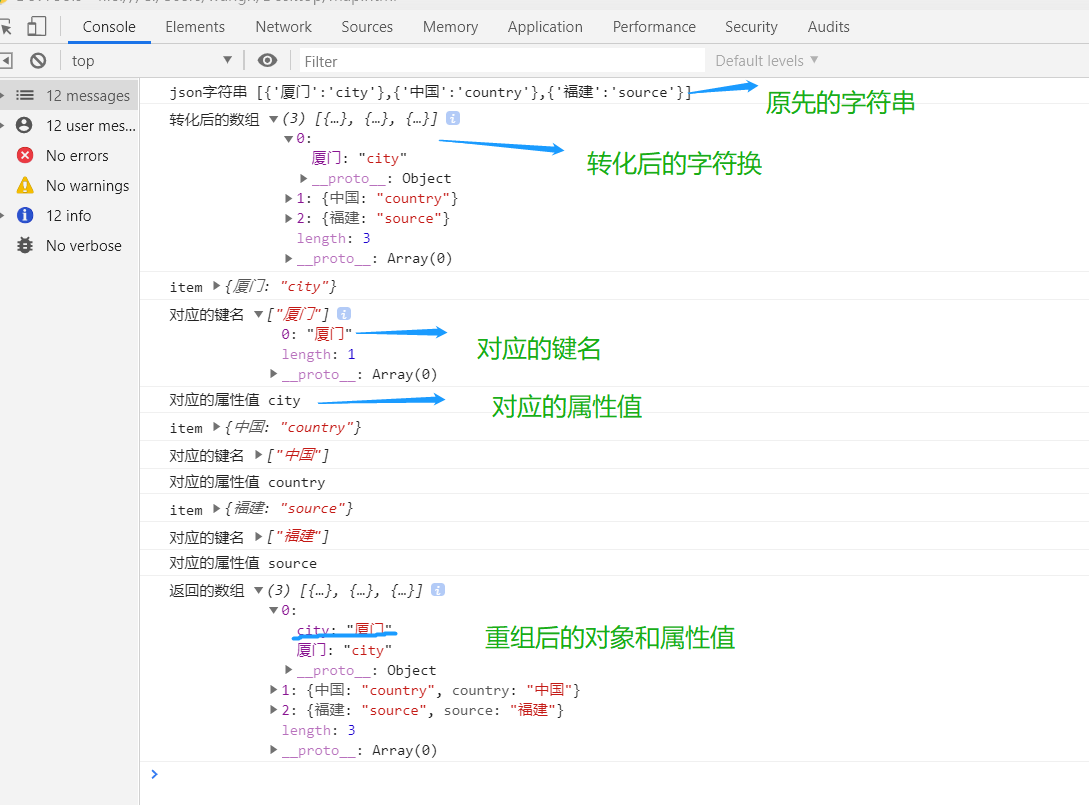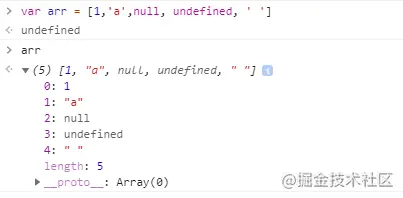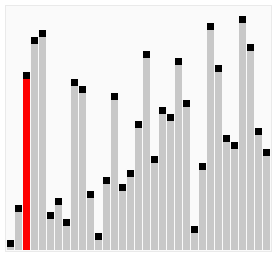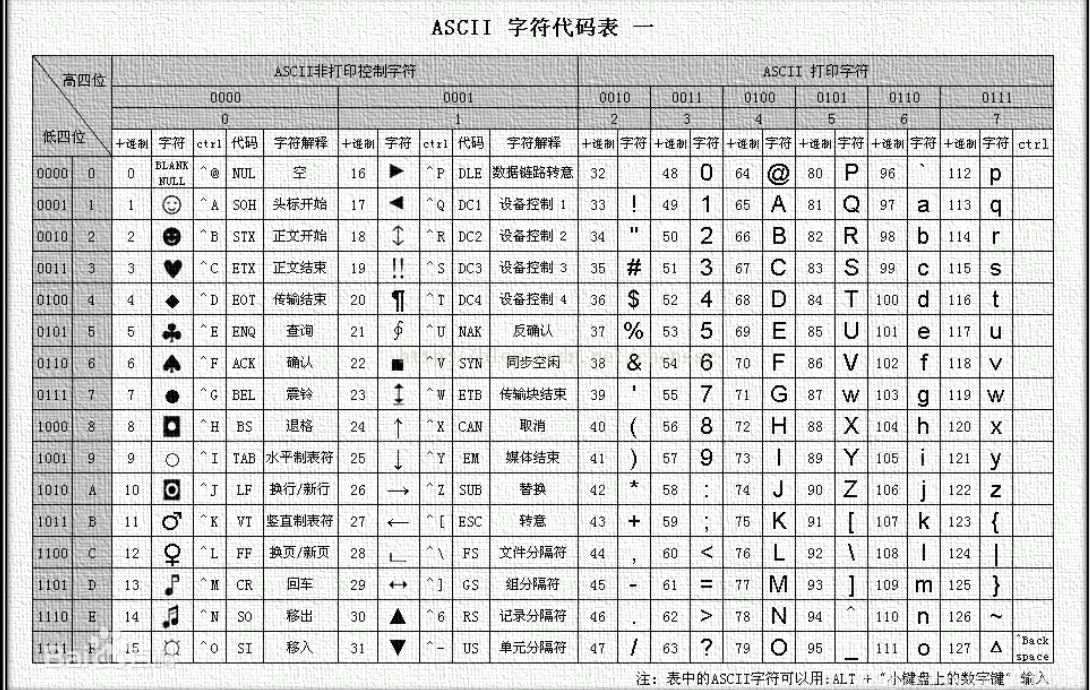# 有趣的JavaScript原生数组函数

+关注继续查看

• 循环：.forEach

• 判断：.some和.every

• 区分.join和.concat

• 栈和队列的实现：.pop, .push, .shift,和 .unshift

• 模型映射：.map

• 查询：.filter

• 排序：.sort

• 计算：.reduce和.reduceRight

• 复制：.slice

• 强大的.splice

• 查找：.indexOf

• 操作符：in

• 走近.reverse.forEach 有一个回调函数作为参数，遍历数组时，每个数组元素均会调用它，回调函数接受三个参数：

• value:当前元素

• index:当前元素的索引

• array:要遍历的数组

1. ['_''t''a''n''i''f'']'].forEach(function (value, index, array) {
2.     this.push(String.fromCharCode(value.charCodeAt() + index + 2))
3. }, out = [])
4. out.join(''
5. // <- 'awesome'

some将会给数组里的每一个元素执行一遍回调函数，直到回调函数返回true。如果找到目标元素，some立即返回true，否则some返回false。回调函数只对已经指定值的数组索引执行；它不会对已删除的或未指定值的元素调用。

1. max = -Infinity
2. satisfied = .some(function (value, index, array) {
3.     if (value > max) max = value
4.     return value < 10
5. })
6. console.log(max)
7. // <- 12
8. satisfied
9. // <- true

.join和.concat 经常混淆。.join(separator)以separator作为分隔符拼接数组元素，并返回字符串形式，如果没有提供separator，将使用默认的,。.concat会创建一个新数组，作为源数组的浅拷贝。

• .concat常用用法：array.concat(val, val2, val3, valn)

• .concat返回一个新数组

• array.concat()在没有参数的情况下，返回源数组的浅拷贝。

1. var a = { foo: 'bar' }
2. var b = [123, a]
3. var c = b.concat()
4. console.log(b === c)
5. // <- false
6. b === a && c === a
7. // <- true

.pop 方法是.push 的反操作，它返回被删除的数组末尾元素。如果数组为空，将返回void 0 (undefined),使用.pop和.push可以创建LIFO (last in first out)栈。

1. function Stack () {
2.     this._stack = []
3. Stack.prototype.next = function () {
4.     return this._stack.pop()
5. Stack.prototype.add = function () {
6.     return this._stack.push.apply(this._stack, arguments)
7. stack = new Stack()
9. stack.next()
10. // <- 3
11. 相反，可以使用.shift和 .unshift创建FIFO (first in first out)队列。
12.
13. function Queue () {
14.     this._queue = []
15. Queue.prototype.next = function () {
16.     return this._queue.shift()
17. Queue.prototype.add = function () {
18.     return this._queue.unshift.apply(this._queue, arguments)
19. queue = new Queue()
21. queue.next()
22. // <- 1
23. Using .shift (or .pop) is an easy way to loop through a set of array elements, while draining the array in the process.
24. list = [1,2,3,4,5,6,7,8,9,10
25. while (item = list.shift()) {
26.     console.log(item)
27. list
28. // <- []

.map为数组中的每个元素提供了一个回调方法，并返回有调用结果构成的新数组。回调函数只对已经指定值的数组索引执行；它不会对已删除的或未指定值的元素调用。

Array.prototype.map 和上面提到的.forEach、.some和 .every有相同的参数格式：.map(fn(value, index, array), thisArgument)

1. values = [void 0nullfalse''
2. values = void 0
3. result = values.map(function(value, index, array){
4.     console.log(value)
5.     return value
6. })
7. // <- [undefined, null, false, '', undefined × 3, undefined]

undefined × 3很好地解释了.map不会对已删除的或未指定值的元素调用，但仍然会被包含在结果数组中。.map在创建或改变数组时非常有用，看下面的示例：

1. // casting
2. [1'2''30''9'].map(function (value) {
3.     return parseInt(value, 10
4. })
5. // 1, 2, 30, 9
6. .map(String.fromCharCode).join(''
7. // <- 'awesome'
8. // a commonly used pattern is mapping to new objects
9. items.map(function (item) {
10.     return {
11.         id: item.id,
12.         name: computeName(item)
13.     }
14. })

filter对每个数组元素执行一次回调函数，并返回一个由回调函数返回true的元素组成的新数组。回调函数只会对已经指定值的数组项调用。

1. [void 0nullfalse''1].filter(function (value) {
2.     return value
3. })
4. // <- 
5. [void 0nullfalse''1].filter(function (value) {
6.     return !value
7. })
8. // <- [void 0, null, false, '']

• a在b之前则返回值小于0

• a和b相等则返回值是0

• a在b之后则返回值小于0

1. [9,80,3,10,5,6].sort()
2. // <- [10, 3, 5, 6, 80, 9]
3. [9,80,3,10,5,6].sort(function (a, b) {
4.     return a - b
5. })
6. // <- [3, 5, 6, 9, 10, 80]

previousValue 是最后一次调用回调函数的返回值，initialValue则是其初始值，currentValue是当前元素值，index是当前元素索引，array是调用.reduce的数组。

1. Array.prototype.sum = function () {
2.     return this.reduce(function (partial, value) {
3.         return partial + value
4.     }, 0
5. };
6. [3,4,5,6,10].sum()
7. // <- 28

1. function concat (input) {
2.     return input.reduce(function (partial, value) {
3.         if (partial) {
4.             partial += ', '
5.         }
6.         return partial + value
7.     }, ''
8. concat([
9.     { name: 'George' },
10.     { name: 'Sam' },
11.     { name: 'Pear' }
12. ])
13. // <- 'George, Sam, Pear'

Array.prototype.slice 能被用来将类数组对象转换为真正的数组。

1. Array.prototype.slice.call({ 0'a'1'b', length: 2 })
2. // <- ['a', 'b']

1. Array.prototype.concat.call({ 0'a'1'b', length: 2 })
2. // <- [{ 0: 'a', 1: 'b', length: 2 }]

1. function format (text, bold) {
2.     if (bold) {
3.         text = '<b>' + text + '</b>'
4.     }
5.     var values = Array.prototype.slice.call(arguments, 2
6.     values.forEach(function (value) {
7.         text = text.replace('%s', value)
8.     })
9.     return text
10. format('some%sthing%s %s'true'some''other''things'

.splice 是我最喜欢的原生数组函数，只需要调用一次，就允许你删除元素、插入新的元素，并能同时进行删除、插入操作。需要注意的是，不同于`.concat和.slice,这个函数会改变源数组。

1. var source = [1,2,3,8,8,8,8,8,9,10,11,12,13
2. var spliced = source.splice(344567
3. console.log(source)
4. // <- [1, 2, 3, 4, 5, 6, 7, 8, 9, 10, 11, 12 ,13]
5. spliced
6. // <- [8, 8, 8, 8]

1. var source = [1,2,3,8,8,8,8,8,9,10,11,12,13
2. var spliced = source.splice(9
3. spliced.forEach(function (value) {
4.     console.log('removed', value)
5. })
6. // <- removed 10
7. // <- removed 11
8. // <- removed 12
9. // <- removed 13
10. console.log(source)
11. // <- [1, 2, 3, 8, 8, 8, 8, 8, 9]

1. var a = { foo: 'bar' }
2. var b = [a, 2
3. console.log(b.indexOf(1))
4. // <- -1
5. console.log(b.indexOf({ foo: 'bar' }))
6. // <- -1
7. console.log(b.indexOf(a))
8. // <- 0
9. console.log(b.indexOf(a, 1))
10. // <- -1
11. b.indexOf(21
12. // <- 1

1. var a = [125
2. 1 in a
3. // <- true, but because of the 2!
4. 5 in a
5. // <- false

1. var a = [376
2. 1 in a === !!a[1
3. // <- true

1. var a = [1178
2. a.reverse()
3. // [8, 7, 1, 1]

.reverse 会修改数组本身。js常见的循环方式以及数组和json字符串之间的转化

0 0JS不能向WASM传递数组？
JS不能向WASM传递数组？
0 0push 是在数组的末尾添加新的数据，数据可以是多个，会依次添加到数组的末尾
0 0js如何精确判断是否是数组？
js如何精确判断是否是数组？
0 0js数组的冒泡排序， 选择排序， 以及快速排序
js数组的冒泡排序， 选择排序， 以及快速排序
0 0js把对象装成数组对象
js把对象装成数组对象
0 00 00 0JavaScript数组的简单排序
JavaScript数组的简单排序 前面的博客分两章分别讲解了数组对象的基本属性和方法，以及数组迭代方法的使用。今天我们再来讲一讲数组的排序问题。 1.数组的sort()方法 说到数组的排序，大家可能第一反应是前面学过一个数组排序的方法sort(),但是使用这个方法时要注意，sort()本身时可以传参数的，如果不传参数，那我们对数组项进行排序时就会按照ASCII字符顺序排序(也可以理解为字典的排序方式)。比如： var arr=["a","A",10,1,20,2]; console.log(arr.sort());//[1,10,2,20,"A","a"] 1
0 0

JavaScript 自学手册文档教程311465

JavaScript入门与实战1889852

jQuery UI 速成58353

Javascript中的函数

Javascript异步编程

JS零基础入门教程（上册）